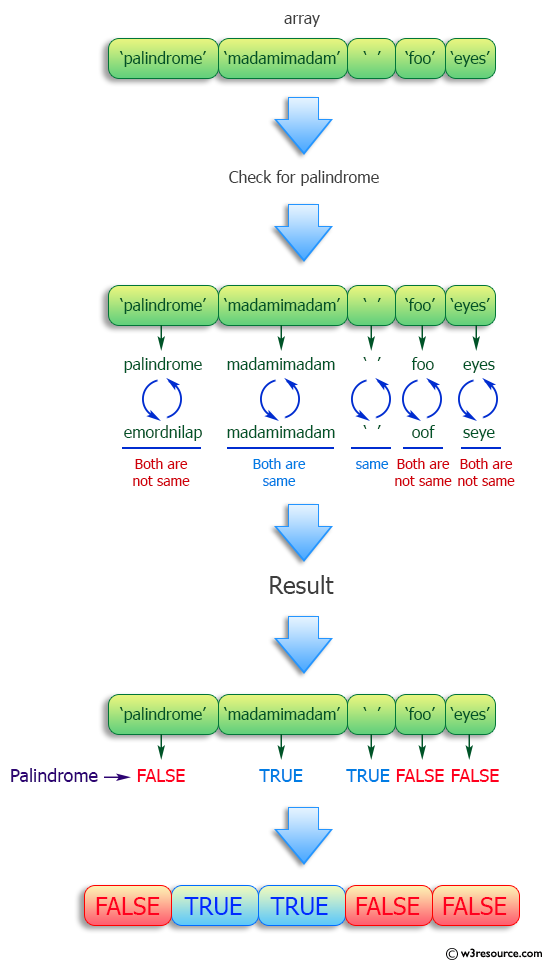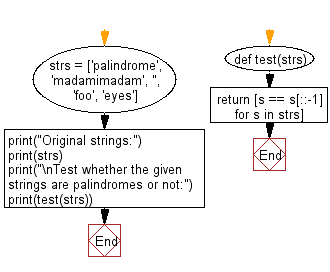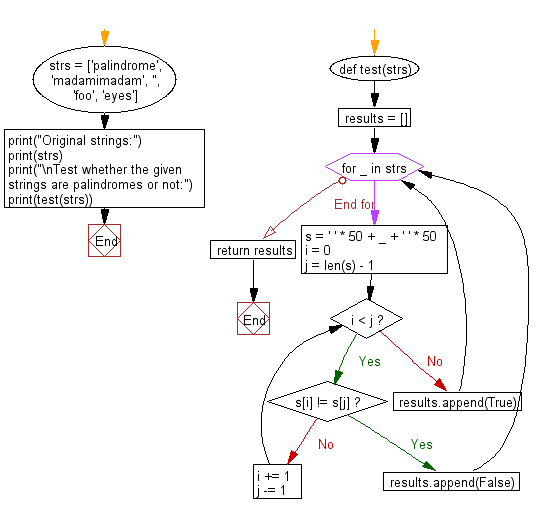﻿ Python: Test whether the given strings are palindromes - w3resource# Python: Test whether the given strings are palindromes

## Python Programming Puzzles: Exercise-12 with Solution

Write a Python program to check whether the given strings are palindromes or not. Return True, False.

```Input:

Output:
[False, True, True, False, False]
```

Pictorial Presentation:Sample Solution-1:

Python Code:

``````#License: https://bit.ly/3oLErEI

def test(strs):
return [s == s[::-1] for s in strs]
print("Original strings:")
print(strs)
print("\nTest whether the given strings are palindromes or not:")
print(test(strs))
``````

Sample Output:

```Original strings:

Test whether the given strings are palindromes or not:
[False, True, True, False, False]
```

Flowchart:## Visualize Python code execution:

The following tool visualize what the computer is doing step-by-step as it executes the said program:

Sample Solution-2:

Python Code:

``````def test(strs):
results = []
for _ in strs:
# padding each string with spaces on both sides
# by default, the pad_to parameter is 50
s = ' ' * 50 + _ + ' ' * 50
i = 0
j = len(s) - 1

while i < j:
if s[i] != s[j]:
results.append(False)
break
else:
i += 1
j -= 1
else:
results.append(True)
return results
print("Original strings:")
print(strs)
print("\nTest whether the given strings are palindromes or not:")
print(test(strs))
``````

Sample Output:

```Original strings:

Test whether the given strings are palindromes or not:
[False, True, True, False, False]
```

Flowchart:## Visualize Python code execution:

The following tool visualize what the computer is doing step-by-step as it executes the said program:

Python Code Editor :

Have another way to solve this solution? Contribute your code (and comments) through Disqus.

What is the difficulty level of this exercise?

Test your Programming skills with w3resource's quiz.

﻿

## Python: Tips of the Day

Clamps num within the inclusive range specified by the boundary values x and y:

Example:

```def tips_clamp_num(num,x,y):
return max(min(num, max(x, y)), min(x, y))
print(tips_clamp_num(2, 4, 6))
print(tips_clamp_num(1, -1, -6))
```

Output:

```4
-1
```